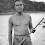### "What on earth is BODMAS"?.

"WHAT on earth is BODMAS"?
Bod is a little person who first appeared on UK Children's TV many years ago. Is BODMAS something to do with Bod and his mothers (Ma's)? - Not really, though it may be a good way to remember BODMAS. It's actually an Acronym (word for letters used to shorten a collection of words to make them more "snappy", for example - did you know that NUT stands for the National Union of Teachers - which may explain why most teachers are NUTs!) sorry Ag,
BODMAS is the secret code which enables us to know exactly the right sequence of doing things mathematically. In particular electronic calculators have to use a rule (known in computing circles as an algorithm) to know which answer to calculate when given a string of numbers to add, subtract, multiply, divide etc.
What do you think the answer to 2 + 3 x 5 is?
Is it (2 + 3) x 5 = 5 x 5 = 25 ?
or 2 + (3 x 5) = 2 + 15 = 17 ?
BODMAS can come to the rescue and give us rules to follow so that we always get the right answer:
(B)rackets
(O)rder
(D)ivision
(M)ultiplication
(A)ddition
(S)ubtraction
According to BODMAS, multiplication should always be done before addition, therefore 17 is actually the correct answer according to BODMAS and will also be the answer which your calculator will give if you type in 2 + 3 x 5 .
I am assuming that you know what everything in BODMAS means apart from "Order". Order is actually a poor word to use here "Power" would be much better though BPDMAS doesn't quite have the same ring to it!
Order means anything raised to the power of a number.
You may have heard of Einstein's famous equation E = mc2 here it can be said that c is raised to the power 2, or c has order 2 or c is squared (they all mean the same thing!).
Here's an example to show how to use all the features of BODMAS:
Explain the answer that a calculator would give to the calculation 4 + 70/10 x (1 + 2)2 - 1 according to the BODMAS rules.
Brackets gives 4 + 70/10 x (3)2 - 1
Order gives 4 + 70/10 x 9 - 1
Division gives 4 + 7 x 9 - 1
Multiplication gives 4 + 63 - 1
Subtraction gives 66

THE PART OF BODMAS MOST OFTEN FORGOTTEN
It is quite common for people to forget that brackets always come first - which means you can calculate the answer of whatever is in the brackets before you attempt to calculate the rest of the problem.
Once you've worked out everything in the brackets, normally these sort of problems become very easy. Provided of course you were born with a brain.Anonymous said…
thanks for the refresher

SabyAnonymous said…
This has got to be the best explanation of BODMAS ever.Vest said…
One of my fav books, Barry Mazur's Imagining numbers,(particularly the square root of minus 15) is a must for the Math Genius.

Wally: If you are reading this, ignore it.